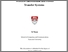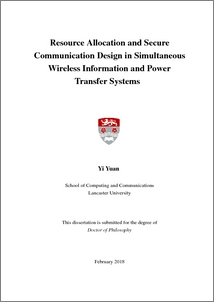# Resource allocation and secure communication design in simultaneous wireless information and power transfer systems

Yuan, Yi (2018) Resource allocation and secure communication design in simultaneous wireless information and power transfer systems. PhD thesis, UNSPECIFIED.Preview
PDF (2018yiyuanphd)
2018yiyuanphd.pdf - Published Version

## Abstract

Radio frequency (RF) energy transfer techniques have been regarded as the key enabling solutions to supply continuous and stable energy for the energy-constrained wireless devices. Simultaneous wireless information and power transfer (SWIPT) has been developed as a more promising RF energy transfer technique since it enables wireless information and wireless energy to access users from a same transmitted signal. Therefore, SWIPT has received remarkable attention. This thesis provides an investigation on applications and security issues of this emerging technology in various wireless communication scenarios. First, this thesis examines the application of SWIPT to a multi-user cooperative network in which the amplify-and-forward (AF) relay protocol is employed at the multi-antenna relay. A power splitting (PS) receiver architecture is utilized at each destination node to implement energy harvesting (EH) and information decoding (ID) simultaneously. The aim of this chapter is to minimize the relay transmit power by jointly designing relay beamforming vectors and PS ratios based on channel uncertainty models. The non-convex problem is converted into a semidefinite programming (SDP) problem by using the semidefinite relaxation (SDR) approach. In addition, a rank-one proof presents that the solution generated by the relaxed problem is optimal to the original problem. Second, a security issue about the SWIPT system is investigated in a cooperative network in the presence of potential eavesdroppers. The AF relay protocol and a PS receiver architecture are adopted at the multi-antenna relay and the desired destination node, respectively. Based on the system setup and the assumption of perfect channel state information (CSI), a transmit power minimization problem combined with the secrecy rate and harvested energy constraints is proposed to jointly optimize the beamforming vector and the PS ratio. The proposed optimization problem is non-convex and hard to tackle due to the issues of the quadratic terms and the coupled variables. To deal with this non-convex problem, two algorithms are proposed. In the first algorithm case, the proposed problem can be globally solved by using a two-level optimization approach which involves the SDR method and the one-dimensional (1-D) line search method. In addition, a rank reduction theorem is introduced to guarantee the tightness of the relaxation of the proposed scheme. In the second algorithm case, the proposed problem can be locally solved by exploiting a low complexity iterative algorithm which is embedded in the sequential parametric convex approximation (SPCA) method. Furthermore, the proposed optimization problem is extended to the imperfect CSI case. Third, a secure communication case is studied in an underlay multiple-input multiple-output (MIMO) cognitive radio (CR) network where the secondary transmitter (ST) provides SWIPT to receivers. In this chapter, two uncertainty channel models are proposed. One is based on the assumption that the ST has the perfect channel knowledge of the secondary information receiver (SIR) and the imperfect channel knowledge of secondary energy receivers (SERs) and primary receivers (PUs). The other one assumes that the ST only has the imperfect channel knowledge of all receivers. In each uncertainty channel model, an outage-constrained secrecy rate maximization (OC-SRM) problem combined with probability constraints is proposed to jointly optimizing the transmit covariance matrix and the artificial noise (AN)- aided covariance matrix. The designed OC-SRM problem for both models is non-convex due to the unsolvable probabilistic constraints. To solve this non-convex problem, the log determinant functions are first approximated to the easy handle the functions that the channel error terms are included in the trace function. Then, the probability constraints are converted into the deterministic constraints by exploiting the Bernstein-type inequality (BTI) approach. Finally, the reformulated problem for both models is solvable by using the existing convex tools. Last, a novel security issue is investigated in a MIMO-SWIPT downlink network where nonlinear energy receivers (ERs) are considered as the potential eavesdroppers. In this chapter, two uncertainty channel models, namely partial channel uncertainty (PCU) and full channel uncertainty (FCU), are proposed. An OC-SRM problem of each model is proposed to design the transmit signal covariance matrix while satisfying probabilistic constraints of the secrecy rate and the harvested energy. To surmount the non-convexity of the proposed OC-SRM problem in each model, several transformations and approximations are utilized. In the PCU model, the OC-SRM problem is first converted into two subproblems by introducing auxiliary variables. Then, three conservative approaches are adopted to obtain the safe approximation expressions of the probabilistic constraints, which are deterministic constraints. Moreover, an alternating optimization (AO) algorithm is proposed to iteratively solve two convex conic subproblems. In the FCU model, log determinant functions are first approximated to the trace functions. Then, the three approaches aforementioned are employed to convert probabilistic constraints into deterministic ones. The bisection method is utilized to solve the reformulated problem. Finally, the computational complexity of the proposed three approaches based on the PCU and FCU model is analyzed.

Item Type:
Thesis (PhD)
Subjects:
ID Code:
123627
Deposited By:
Deposited On:
23 Feb 2018 10:12
Refereed?:
No
Published?:
Unpublished